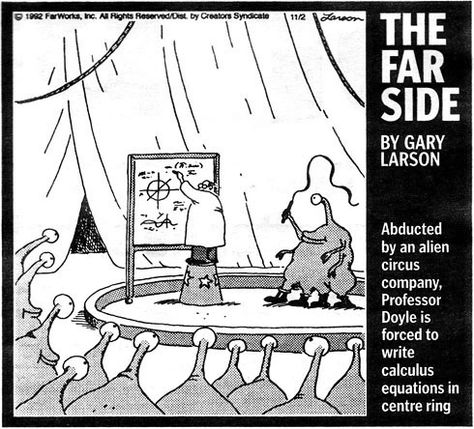# Differential and Integral Calculus 2T (104013)

Department of Mathematics

Lecturer: Samy Zafrany

## Lecture Notes

Corrections and improvement suggestions are most welcome (send to email above).

## extended Spherical Harmonics 3D surface with 12 varying parameters

$r = \sin^{m_1}(m_0\phi) + \cos^{m_3}(m_2\phi) + \sin^{m_5}(m_4\theta) + \cos^{m_7}(m_6\theta) + \sin^{m_9}(m_8\theta) + \cos^{m_{11}}(m_{10}\theta) \\$
The parametric equations are: $\begin{cases} x &= r \sin(\phi) \cos(\theta) \\[0.5em] y &= r \cos(\phi) \\[0.5em] z &= r \sin(\phi) \sin(\theta) \end{cases}$ Initial values of the twelve parameters are: $\newcommand\T{\Rule{0pt}{1em}{.3em}}$ $\renewcommand{\arraystretch}{0.9} \begin{array}{|c|c|c|c|c|c|c|c|c|c|c|c|} \hline m_0 & m_1 & m_2 & m_3 & m_4 & m_5 & m_6 & m_7 & m_8 & m_9 & m_{10} & m_{11} \\ \hline 4.0 & 3.0 & 2.0 & 3.0 & 6.0 & 2.0 & 6.0 & 4.0 & 8.0 & 4.0 & 6.0 & 8.0 \\ \hline \end{array}$

The Python code for generating this movie can be obtained from here.

## Classic Spherical Harmonic 3D surface (moving perspectives)

This is the classical Spherical Harmonics 3D surface with 8 static parameters

$r = \sin^{m_1}(m_0\phi) + \cos^{m_3}(m_2\phi) + \sin^{m_5}(m_4\theta) + \cos^{m_7}(m_6\theta) \\$
The parametric equations are: $\begin{cases} x &= r \sin(\phi) \cos(\theta) \\[0.5em] y &= r \cos(\phi) \\[0.5em] z &= r \sin(\phi) \sin(\theta) \end{cases}$ Static values of the eight parameters are: $\newcommand\T{\Rule{0pt}{1em}{.3em}}$ $\renewcommand{\arraystretch}{0.9} \begin{array}{|c|c|c|c|c|c|c|c|} \hline m_0 & m_1 & m_2 & m_3 & m_4 & m_5 & m_6 & m_7 \\ \hline 4.0 & 3.0 & 2.0 & 3.0 & 6.0 & 2.0 & 6.0 & 4.0 \\ \hline \end{array}$

The Python code for generating this movie can be obtained from here.

## Conic Section 3D Animation

The Cartesian equation of the Conic Section surface is given by $\frac{x^2}{a^2} + \frac{y^2}{b^2} = \frac{z^2}{c^2}$

## 3D Two-Sheeted Hyperboloid with a tangent plane

The Cartesian equation of the Hyperoloid surface is given by $x^2 - y^2 - z^2 = 4$ The tangent plane is $3x - 2y - z - 4 = 0$ The tangent point is $(3,2,1)$.

## Divergence and curl | Fluid flow with complex functions, part 1